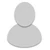## FANDOM

10,083 Pages

• I thought of this game idea on Discord, so anyways I'll post it here.

So you say some intensity, and the next person has to estimate the equivalent pressure of the wind speed you give them.

Example:

Person Starting Game: What would the 160 mph equivalent of 90/973 be?

Next person: 160/919, what would be the 75 mph equivalent of 160/940 be?

Next person: 75/995, what would be the 130 mph equivalent of 175/902 be?

And it continues. You can say a storm name like "What would the 160 mph equivalent of Olga'01 peak be?" instead of saying "What would the 160 mph equivalent of 90/973 be?"

Alright, let's begin.

What would the 160 mph equivalent be of Bob'85? (75/1002)

• 160/942, what would be the 100 mph equivalent be of Edith '71? (160/943)

•